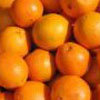#### You may also like### Weighing Fruit

Can you use this information to estimate how much the different fruit selections weigh in kilos and pounds?

# At the Pumps

##### Age 7 to 11 Challenge Level:

We had about 40 correct answers for this problem. Some of which are set out below. Beth used her good method for finding 12 times by breaking it down - well done! Shannon set it out all very logically to get the correct answer. Dana and one or two others said how they'd used the internet to find information. Ben's answer was different because a gallon measure is different in USA. I included Joe's as it is the first I've seen coming from the islands off Scotland. Finally, Abidine showed how to use many places of decimals and come up with a correct answer.

Well done all of you!

Beth
I found out that there was 4.5 litres in a gallon.
Say one litre cost £1.00,
How much would it cost if you needed to fill a 12 gallon petrol tank?
So one gallon costs £4.50p, two gallons cost £9.00, three gallons cost £13.50p, six gallons would cost £27.00, twelve gallons would cost a whopping £54.00.
Easy peasy lemon squeesy

Shannon
First I found out how many litres in a gallon and I came up with 4.55 litres.
Then I multiplied 4.55l by 12 to see how many litres were in 12 gallons and I got the answer of 54.60 litres.
Then I multiplied 54.60 litres by £1 and got the answer of £54.60

Dana
First I went on google and typed in how many liters are in 1 gallon and my answer from that was 4.5
So I added that 12 times and my answer from that was 54.0 so we put that in a money way and it was £54.00

Ben
1 gallon = 3.785 litres
12 gallons = (3.785 x 12) litres
(3.785 x 12) = 45.42
12 gallons = 45.42 litres
1 litre = £1
therefore 12 gallons cost £45.42

Joe
First of all I found out how many litres there are in a gallon > 4.55 then multiplied that by 10 > £45.50 then multiplied by 2 > 9.10
then I added them together > £54.60

Abidine
Well, I found out that 1 gallon contains 4.54609188 litres.
Therefore, to fill my 12 gallon petrol tank I had to multiply 4.54609188 by 12. That equals 54.55310256. with each litre costing £1.00, that meant you had to get the 4 most significant figures (pounds and pence), which is £54.55.